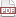### Multiple imputation

26 September 10. [link]PDF version

Imputation is the statistician's term for what everybody else calls filling in missing data. In some contexts, imputation is just a part of the background routine, whether you're willing to admit it or not. Are you giving the mean of a set of observations and just ignoring the missing values? That's equivalent to imputing each missing value as having the value of the mean. The expected value is expected to be right, but the variance for a list of 100 items where ten were filled in at the mean is smaller than the variance for a list of 90 known items (which is what results from listwise deletion). Which is the right variance? We can't answer that question with the information to this point, because we don't have a model of why the missing data is missing or how it differs from the rest of the data.

To fully flesh out the situation, we'll need two models: one model that is the data generation story we want to tell, like a linear regression story or draws from a Multivariate Normal distribution, and the other model is the one that explains how we filled in the missing data. That model is probably not much like the model that you intend to estimate; it is probably much simpler, like a plain Multinomial distribution. After you make a filling-in-the-blanks draw from the distribution, you can find the variance of the statistic you want for the overall distribution based on the now-complete data; and for several draws from the fill-in distribution, you can find the variance of that statistic across data sets.

I chose that within/across language to parallel the language of within group/across group variance calculations from the ANOVA world, because the process here is analogous to the process there.

That's the whole story: specify a model by which your data was generated, then use that model to fill in a series of data sets, calculate your preferred statistic for each, and compute total variance as the sum of within-imputation and across-imputation variance.

###### ¿Is it Bayesian?

Really, the storyline is just the convolution of two models. Let the parent model be a distribution over the outcome f (out| d, md ), where md is missing data, and let md have distribution g(md ). To observe the final outcome of the model, you'll need to find the convolution, fog, which, keeping to blog-level notational precision and taking the observed data as having probability one, one could write as the overall joint distribution f (out, d, md )= f (out| d, md )g(md ) At this point, it should start looking like Bayes's rule as presented in the typical Bayesian updating setup. The coincidence here is that both Bayes's rule and the missing data are convolutions of two models, and therefore both will take this form.

That's convenient because our Bayesian friends have been spending the last few decades putting real work into developing the computational tools one would require for convoluting two probability distributions. So if you wanted to, you could think of the missing data problem as a Bayesian problem, pull out your generalized Bayesian solver (which probably means your MCMC routine), and get results. If you don't have a generalized solver, you can set up your missing data models so that the two distributions are from the table of conjugate distributions, and calculate results for the output distribution with pencil and paper.

The direction you take and tools you use probably depends on what you want your output to be. If you want the entire distribution, then a Bayesian-style technique is your only bet. If you just want total variance for a statistic, then the full convolution is overkill, and the method I mentioned at the head of this entry, where you simply generate a half-dozen full data sets and sum up the within/across variances, will give you the most accuracy for your computational buck.

There are several choices in naming a setup: the context, the methods used, et cetera. The real crux of the missing data problem is in specifying an auxiliary model for the missing data to accompany your main model, making it just one of a wide range of methods that join two complementary models. But in the textbooks, you'll find the process named after the method of calculating the post-convolution statistic's variance, multiple imputation, although there are other methods to doing the model-merging calculations.

Next time: the social implications.